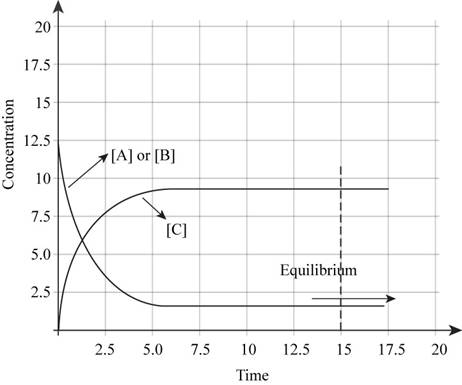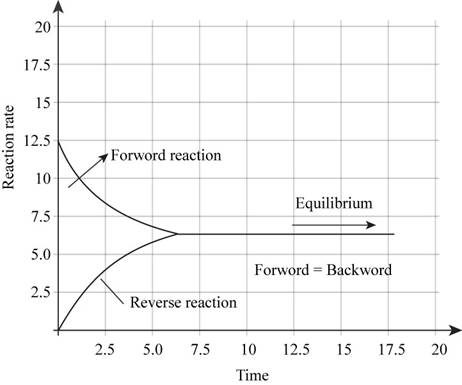# Characterize a system at chemical equilibrium with respect to each of the following a . the rates of the forward and reverse reactions b . the overall composition of the reaction mixture For a general reaction 3 A ( g ) + B ( g ) → 2 C ( g ) , if one starts an experiment with only reactants present, show what the plot of concentrations of A, B, and C versus time would look like. Also sketch the plot illustrating the rate of the forward reaction and rate of the reverse reaction versus time.### Chemistry: An Atoms First Approach

2nd Edition
Steven S. Zumdahl + 1 other
Publisher: Cengage Learning
ISBN: 9781305079243### Chemistry: An Atoms First Approach

2nd Edition
Steven S. Zumdahl + 1 other
Publisher: Cengage Learning
ISBN: 9781305079243

#### Solutions

Chapter 12, Problem 1RQ
Textbook Problem

## Characterize a system at chemical equilibrium with respect to each of the followinga. the rates of the forward and reverse reactionsb. the overall composition of the reaction mixture For a general reaction 3 A ( g ) + B ( g ) → 2 C ( g ) , if one starts an experiment with only reactants present, show what the plot of concentrations of A, B, and C versus time would look like. Also sketch the plot illustrating the rate of the forward reaction and rate of the reverse reaction versus time.

Expert Solution
Interpretation Introduction

Interpretation: The given systems at equilibrium are to be characterized. For the given reaction, the required plot is to be shown. The plot that illustrates the rate of forward reaction and rate of reverse reaction is to be sketched.

Concept introduction: Chemical equilibrium is a state of a system in which the rate of forward reaction and that of the backward reaction is equal. It is affected by various factors such as concentration of reactants or products, temperature, pressure.

1. a) Rate of the forward and reverse reactions are equal at equilibrium.
2. b) The overall composition remains constant at equilibrium.

The plot of concentrations of A,B and C versus time is shown in Figure 1.

The plot illustrating the rate of forward reaction and rate of reverse reaction versus time is shown in Figure 2.

### Explanation of Solution

Explanation

(I)

(a)

To determine: The characterization of the given system at equilibrium.

The rate of the forward and reverse reactions is equal at equilibrium.

At equilibrium, the concentrations of reactants and products do not change. When equilibrium is attained by a system, equilibrium rate of forward reaction will be equal to rate of backward reaction.

(b)

To determine: The characterization of the given system at equilibrium.

The overall composition remains constant at equilibrium.

The forward and backward reaction at equilibrium proceeds with same rate, hence the concentration of reactants and products do not change. Therefore, the overall composition remains constant at equilibrium.

(II)

To determine: The plot of concentrations of A,B and C versus time and the plot illustrating the rate of forward reaction and rate of reverse reaction versus time.

The plot of concentrations of A, B and C versus time is shown in Figure 1.

The given reaction is,

3A(g)+B(g)2C(g)

If one starts with only reactants present, then the plot of concentrations of A,B and C versus time will be like,Figure 1

In the beginning of the reaction, the concentration of reactants is more. As the time proceeds, the concentration of reactants decreases and the concentration of products increases. At equilibrium, there is no change in the concentrations of reactants and products.

The plot illustrating the rate of forward reaction and rate of reverse reaction versus time is shown in Figure 2.

In the beginning of the reaction, the concentration of reactants is more. Therefore, rate of forward reaction is high. But as the reaction proceeds further, the reactants are consumed to form products. Hence rate of forward reaction decreases and rate of backward reaction increases. At equilibrium, both the rates are equal. Therefore, plot illustrating the rate of forward reaction and rate of reverse reaction versus time will be like,Figure 2

Conclusion

Conclusion

1. a) Rate of the forward and reverse reactions are equal at equilibrium.
2. b) The overall composition remains constant at equilibrium.

The plot of concentrations of A, B and C versus time is shown in Figure 1.

The plot illustrating the rate of forward reaction and rate of reverse reaction versus time is shown in Figure 2

### Want to see more full solutions like this?

Subscribe now to access step-by-step solutions to millions of textbook problems written by subject matter experts!

### Want to see more full solutions like this?

Subscribe now to access step-by-step solutions to millions of textbook problems written by subject matter experts!